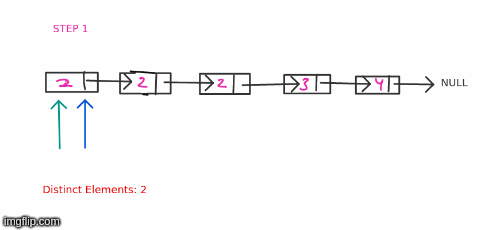# Printing distinct elements in a sorted linked list

Given a linked list sorted in ascending order, print only the distinct elements in the linked list with only one pass through the linked list and without using more than ` O(1) ` space.

#### Example Test Cases

##### Sample Test Case 1

Input Linked List: Given a linked list like this `1->2->2->3->3->4 `
Expected Output: `1->2->3->4 ` (since, there are 4 distinct values in the linked list). Only the first 4 elements need to be outputted.

##### Sample Test Case 2

Input Linked List `5->8->9->9->10 `
Expected Output: `5->8->9->10`

#### Solution

Since the array is sorted, duplicate numbers will occur together at consecutive positions, and we need to choose only one of them. Therefore, to solve this problem 2 pointer method can be used.

We can create one fast pointer which moves by 1 element at each step one slow pointer which moves only when the fast pointer moves on to a new element. Now, whenever the values of fast pointer and slow pointer differ we print the distinct value and move the slow pointer to the location of fast pointer.

Take a look below:#### Implementation

```#include <bits/stdc++.h>
using namespace std;

typedef struct node {
int data;
node* next;
} node;

/* Initially HEAD is null because list is empty */
node* HEAD = NULL;

// Inserts a node with data at the end of current linked list
void insertAtTheEnd(int data) {

/* Step 1: Create a New Node dynamically by using new operator */
node* nodeToBeInserted = new node;
nodeToBeInserted->data = data;
nodeToBeInserted->next = NULL; /* Since, the node is getting inserted at the last, it's next will be NULL */

/* If head is NULL, then this is the first node that is getting inserted */
if (HEAD == NULL) {
}
else {
/* At the end of this loop, curr will point to the last node */
node* curr = HEAD;
while (curr->next != NULL) {
curr = curr->next;
}
/* Attach the nodeToBeInserted to the end of last node (curr) */
curr->next = nodeToBeInserted;
}
}

void printList() {
node* curr = HEAD;
while (curr != NULL) {
cout << curr->data << "\n";
curr = curr->next;
}
}

void printOnlyDistinct() {
/* Create two pointers, slow and fast pointer */
node* sp = HEAD, *fp = HEAD;
while (fp != NULL) {
if (sp == fp) {
cout << sp->data << "\n";
}
fp = fp->next;
if (fp != NULL && fp->data != sp->data) {
sp = fp;
}
}
}

int main() {

/** Creating the linked list */
insertAtTheEnd(5);
insertAtTheEnd(10);
insertAtTheEnd(10);
insertAtTheEnd(15);
insertAtTheEnd(15);

/** Printing all the elements of the list */
cout << "Printing all the elements\n";
printList();

/** Printing only the distinct elements of the list */
cout << "Printing only distinct elements \n";
printOnlyDistinct();

return (0);
}```

Scroll to Top
[gravityforms id="5" description="false" titla="false" ajax="true"]